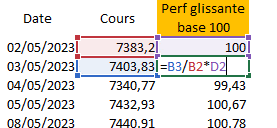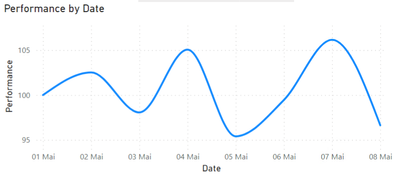cancel
Showing results for
Did you mean:

Fabric is Generally Available. Browse Fabric Presentations. Work towards your Fabric certification with the Cloud Skills Challenge.New Member

## Rolling performance measure

Hello everyone,

I'd like to ask you about a little problem I'm having with Power Bi.

I want to create a dynamic graph with the following inputs: dates (x-axis) and sliding performance (y-axis) calculated via a measure.

My problem is with the measure. Using the latter, I want to calculate the performance of an index between 2 dates that can be modified.

The first performance data (the one with the earliest date in relation to the dates selected via the slicer) must always be 100. The other data are calculated as follows (see calculation below and photo of calculation):

(Today's price) / (Last working day's price) * (Last working day's performance)

The problem is that I don't know how to do this using a measure. Does anyone have a solution or any ideas I can explore?

Below are screenshots of what I want to display and the data I'm using.1 ACCEPTED SOLUTIONPost Prodigy

``````Performance =
VAR _MIN =
CALCULATE(
MIN('Calendar'[Date]),
ALLSELECTED('Calendar'[Date]) )

VAR _MAX =
CALCULATE(
MAX('Calendar'[Date]),
ALLSELECTED('Calendar'[Date]) )

VAR _MIN_Value = CALCULATE(
[SUM Course],
'Calendar'[Date] = _MIN)

VAR _MAX_Value = CALCULATE(
[SUM Course],
'Calendar'[Date] = _MAX)

VAR _DIVIDE = DIVIDE([SUM Course], _MIN_Value) * 100

RETURN
IF(
HASONEVALUE('Calendar'[Date]),
_DIVIDE)``````
9 REPLIES 9Post Prodigy

So you have the smalest date selected by a slicer = Date de debut?
And then you want compare another (random) date selected by a slicer to this earliest date = Date de fin?

Did I get it right?
How do you select these dates?New Member

Hi Sergej,

You can see (with the screen on my comment) the date selector I use on Power Bi.Post Prodigy

Ah ok,
so it is a simple date slicer with a slider.
So you define MIN and MAX dates for your purpose.
Right?New Member

Yes exactlyPost Prodigy

sthg like this?After change your MIN Date using a slicer it will look like this:
New reference value is then your new MIN Date selected.That´s what you wanted to see?

Line Chart:Post Prodigy

``````Performance =
VAR _MIN =
CALCULATE(
MIN('Calendar'[Date]),
ALLSELECTED('Calendar'[Date]) )

VAR _MAX =
CALCULATE(
MAX('Calendar'[Date]),
ALLSELECTED('Calendar'[Date]) )

VAR _MIN_Value = CALCULATE(
[SUM Course],
'Calendar'[Date] = _MIN)

VAR _MAX_Value = CALCULATE(
[SUM Course],
'Calendar'[Date] = _MAX)

VAR _DIVIDE = DIVIDE([SUM Course], _MIN_Value) * 100

RETURN
IF(
HASONEVALUE('Calendar'[Date]),
_DIVIDE)``````New Member

Yes this is exactly what I'm looking for ! thank you so much !!!Post Prodigy

You are welcome.
Have a nice weekend.New Member

Thanks ! Have a nice weekendAnnouncements#### Power BI Monthly Update - November 2023

Check out the November 2023 Power BI update to learn about new features.#### The largest Power BI and Fabric virtual conference

130+ sessions, 130+ speakers, Product managers, MVPs, and experts. All about Power BI and Fabric. Attend online or watch the recordings.Top Solution Authors
Top Kudoed Authors
Users online (6,140)